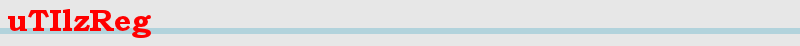Index uTIlzreg - uTIlzReg_LDDMM_Beg - uTIlzReg_GeoShoot - uTIlzReg_Demons - uTIlzReg_Tools

uTIlzReg is for research purposes only and has not been approved for clinical use.

### Presentation:

uTIlzReg_Tools is set of helpful tools to treat or analyse nifti images.

### Calling the function:

./uTIlzReg_Tools <options>

### Options:

#### ARITHMETIC ON IMAGES:

-weightedSum [Nb Images] [Weight1][Image1] [Weight2][Image2]... [Output Image]
-> Compute the weighted sum of one or several images

-weightedMean [Nb Images] [Weight1][Image1] [Weight2][Image2]... [Output Image]
-> Compute the weighted mean of several images

-weightedStd [Nb Images] [Weight1][Image1] [Weight2][Image2]... [Output Image]
-> Compute the weighted standard deviation between several images

-NormGrad [ImageIn][ImageOut]
-> Compute the norm of the gradients of [ImageIn] to detect the boundaries. Result is stored in [ImageOut].

#### IMAGE TREATMENT:

-AnisoDiff [ImageIn][ImageOut] [ax][ay][az] [IterationNb][dTau]
-> Perform anisotropic diffusion of [ImageIn]. Spatial diffusion parameters are [ax][ay][az] and temporal (iteration more precisely) parameters are [IterationNb][dTau]. Result is stored in [ImageOut].

-Diffusion [ImageIn][ImageOut] [IterationNb][dTau] <[Mask][MaskId]>
-> Perform isotropic diffusion of [ImageIn]. Spatial diffusion parameter is 1 and temporal (iteration more precisely) parameters are [IterationNb][dTau]. Result is stored in [ImageOut]. If the options <[Mask][MaskId]> are defined, the diffusion is only performed where the intensities of are equal to [MaskId].

-Erosion [ImageIn][ImageOut] [IterationNb] <[Mask][MaskId]>
-> Erosion of [ImageIn] during [IterationNb] iterations and with a structuring element of 3*3*3 voxels. Result is stored in [ImageOut]. If the options <[Mask][MaskId]> are defined, the erosion is only performed where the intensities of are equal to [MaskId].

-Dilation [ImageIn][ImageOut] [IterationNb] <[Mask][MaskId]>
-> Dilation of [ImageIn] during [IterationNb] iterations and with a structuring element of 3*3*3 voxels. Result is stored in [ImageOut]. If the options <[Mask][MaskId]> are defined, the dilation is only performed where the intensities of are equal to [MaskId].

-PutGLInROI [ImageIn][ImageOut] [Rx1][Rx2][Ry1][Ry2][Rz1][Rz2] [GreyLev]
-> Put the grey level [GreyLev] in the ROI defined by [Rx1][Rx2][Ry1][Ry2][Rz1][Rz2] (in voxels)

-MaskImage [ImageIn][ImageOut] [Mask][MaskId]
-> Apply the mask [Mask] to image [ImageIn]. The values at [MaskId] in [Mask] are set to zero in [ImageOut]

-RemoveSmallReg [ImageIn][ImageOut] [IdVoxShape][IdVoxBackG] [MaxSize]
-> Put to [IdVoxBackG] the connected sets of voxels of intensity [IdVoxShape] having a size of less than [MaxSize] voxels.

-GreyLevThresh [ImageIn][ImageOut] [NewMinGL][NewMaxGL]
-> Set to [NewMinGL] all the grey levels under [NewMinGL] and to [NewMaxGL] all the grey levels above [NewMaxGL]. Remark: if [NewMinGL]==[NewMaxGL] a traditional threshold around [NewMinGL] is done instead.

-GreyLevResample [ImageIn][ImageOut] [NewMinGL][NewMaxGL]
-> Linearly Resample the grey levels between [NewMinGL] and [NewMaxGL].

#### TREATMENT OF DEFORMATIONS AND IMAGE SPACES:

-UnderSample [ImageIn][ImageOut] [factor]
-> Undersample the resolution of [ImageIn] with a factor [factor]. The result is saved in [ImageOut].

-RegCenterImage [MovingIma][FixedIma][TransfoIma]
-> Transform the 'image 2 world' properties of [ImageIn] to be centered on [ImageOut]. Result is in [TransfoIma].

-TransfoImag [SourceImage] [DF_X][DF_Y][DF_Z] [TransfoImage]
-> Transform the image [SourceImage] using the displacement field [DF_X][DF_Y][DF_Z] (in mm). The transformed image [TransfoImage] is in the D.F. coord. system.

-ComposeDispFields [DF1_X][DF1_Y][DF1_Z] [DF2_X][DF2_Y][DF2_Z] [DFC_X][DC1_Y][DC1_Z]
-> Compute DispField1 o DispField2 (the displacement fields are in mm / DispFieldComp <- coord. sys. of DispField2)

-RemAffineContribDF [DFi_X][DFi_Y][DFi_Z] [r_xx r_xy r_xz t_x r_yx ... t_z] [DFo_X][DFo_Y][DFo_Z]
-> Suppose [DFi] = (Affine def.) o (Non-affine def.) -> remove the affine contrib [r_xx...] and save the result in [DFo]

#### ANALYSE IMAGES AND DEFORMATIONS:

-SSD [Image1] [Image2] <[Margin]>
-> Measure the sum of squared differences and the mutual information between two images

-Overlap [Image1] [Image2] [Thresh] <[Margin]>
-> Compute de overlap (Dice metric) between two images. In each image, the shape is the grey levels strictly above [Thresh].

-Surf_Av_Std_values [RefIma] [Thresh] [Values]
-> Mesure the average and std of the intensities in [Values] at the surface of [RefIma] at the threshold [Thresh]

-ROI_Av_Std_values [Image] [Rx1][Rx2][Ry1][Ry2][Rz1][Rz2]
-> Mesure the average and std of the intensities in the ROI of [Image] defined by [Rx1][Rx2][Ry1][Ry2][Rz1][Rz2]

-DispFieldStudy [DefX][DefY][DefZ] <[Mask][MaskID]>
-> Quantify the properties of the desiplacement field [DefX][DefY][DefZ]. If the options <[Mask][MaskId]> are defined, the properties are only studied where the intensities of are equal to [MaskId].

-CompareLandmarks [Target lm file] [DefX][DefY][DefZ] [Source lm file]
-> Measure how the displacement field [DefX][DefY][DefZ] matches the target landmarks [Target lm file] to the source ones of [Source lm file]

Main menu